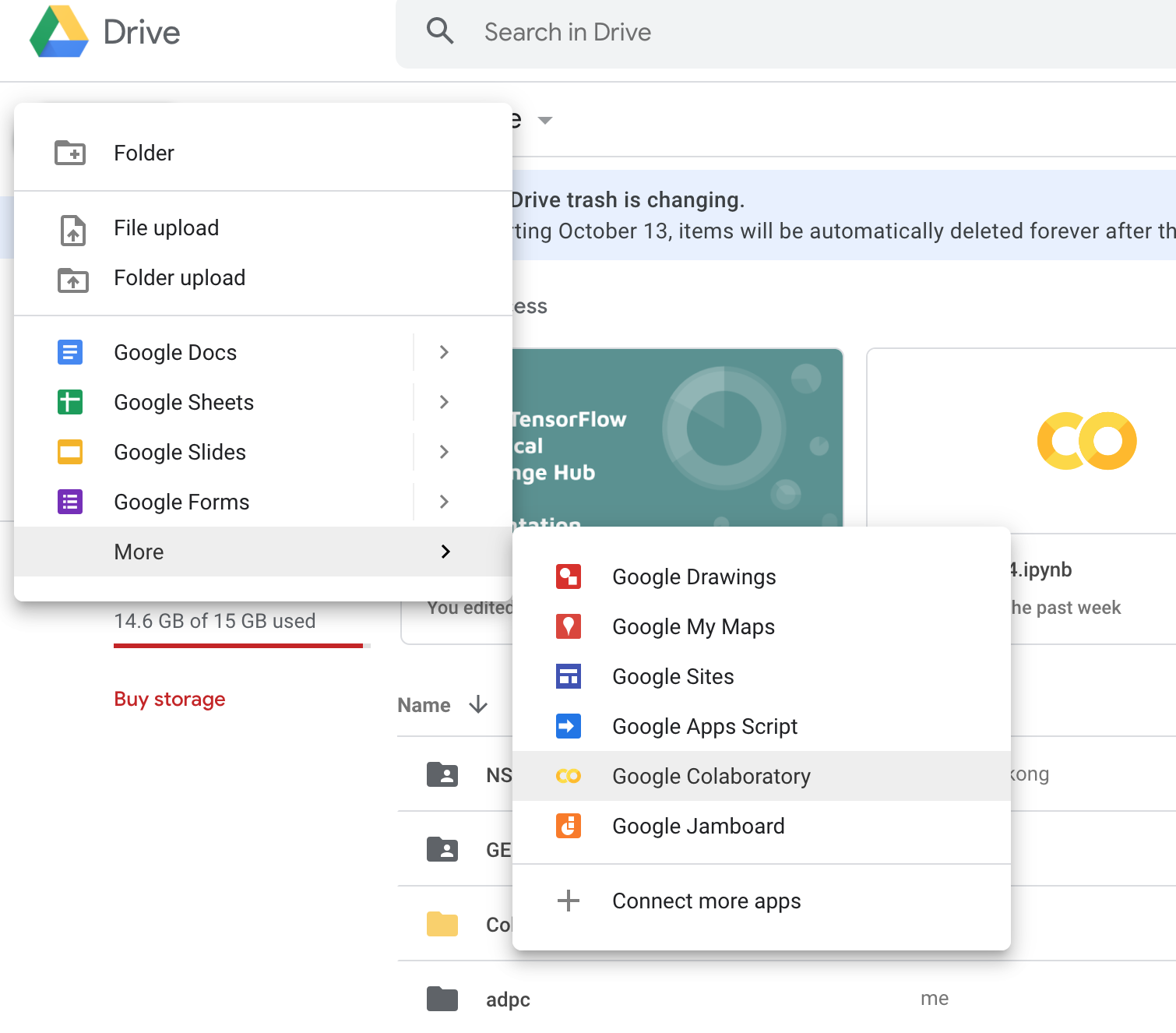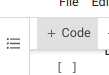# Rice Mapping using machine learning in Colab

Machine learning using the Google Earth Engine python API

Step 1: open a notebookStep 3: add the line below and hit run. This will install the earthengine map library

```!pip install geemap
```

Step 4: add the line below to install the geemap package and authenticate and initialize Earth Engine.

```# Installs geemap package
import geemap.eefolium as geemap
# Authenticates and initializes Earth Engine
import ee
ee.Authenticate()
ee.Initialize()
```

Step 5: To import data from Google Earth Engine Asset and assign value for primitives, add the below code to colab!

```#Import Data from Google Earth Engine Asset
composites = ee.ImageCollection("projects/servir-mekong/yearlyComposites");
data = ee.FeatureCollection("projects/servir-mekong/referenceData/riceTraining");

# Select rice and no rice
rice = data.filter(ee.Filter.eq('land_class',1))
non_rice = data.filter(ee.Filter.eq('land_class',0))
```

Step 6: Show rice and non_rice reference points data in geemap

```Map = geemap.Map(center=[12,105], zoom= 8)
Map

```

Step 7: Sample training sample for 2018 on our servir-regional composite first. After that, train the samples in Random Forest algorithm to create primitive with the following code.

```# Sample the composite
y = 2018;
start = ee.Date.fromYMD(y,1,1);
end = ee.Date.fromYMD(y,12,31);
image = ee.Image(composites.filterDate(start,end).first()).divide(10000);

trainingData  = data.filter(ee.Filter.eq("year",y));

trainingSample = image.sampleRegions(collection=trainingData,properties=["land_class"],scale=30,geometries=True);
print("Training Sample " , trainingSample.size().getInfo())

#Run the Random Forest model and show the map
bandNames = image.bandNames();
classifier = ee.Classifier.randomForest(25,0).setOutputMode('PROBABILITY').train(trainingSample,"land_class",bandNames);
classification = image.classify(classifier).multiply(100).clip(cambodia)
```

Step 8: Copy the below code to show the rice primitive layer in geemap.

```Map = geemap.Map(center=[12,105], zoom= 8)
Map

```

Find the full colab script here

1.Marcelo Brito says:

Hi! Thank you for this excellent tutorial.
I have a question: how do I collect my training samples? and how can i store them in a way that i can use in gee?

1.thisearthsite says:

you could use Collect Earth to collect point data : https://collect.earth/

2.Muhammad Iqbal Habibie says:

I try to follow the step. I have problem on step 7. There is an error message like this :

—————————————————————————
HttpError Traceback (most recent call last)
/usr/local/lib/python3.7/dist-packages/ee/data.py in _execute_cloud_call(call, num_retries)
329 try:
–> 330 return call.execute(num_retries=num_retries)

5 frames
HttpError:

During handling of the above exception, another exception occurred:

EEException Traceback (most recent call last)
/usr/local/lib/python3.7/dist-packages/ee/data.py in _execute_cloud_call(call, num_retries)
330 return call.execute(num_retries=num_retries)